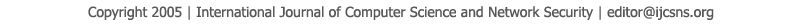To search, Click below search items.All Published Papers Search Service
 Title Author Year Keyword
 Title The Dynamic Dual Key Encryption Algorithm Based on joint Galois Fields Author Abdul Monem S. Rahma, Basima Z.Yacob Citation Vol. 11  No. 8  pp. 190-199 Abstract This paper presents a novel symmetric approach (algorithm) called Dynamic Dual Key Encryption Algorithm which uses dual key for encryption with variable (dynamic) block bits size, where each block of size 3, 4, 5 and 6 bits are interpreted as an element of a finite field. The first key is called control key determines the length of bits block(3, 4, 5, or 6bits block) size to encrypt, and the second key is used for encryption by using an equation Y=XA+B Where X is bits block, A and B are the encryption keys. The mathematical operations addition and multiplication in this equation are based on mathematical theory of Galois field GF. The proposed algorithm achieves best results, where it provides high level of security by using dual key and four dynamic tiny block cipher to decrypt the ciphertext with 128 bits, the attacker needs 1.86285884e+204 of possibilities of keys as minimum and 1.80032832e+399 as maximum, In addition, it is approximately 43 times faster than AES encryption and 64 times faster than AES decryption. Keywords Dynamic block, Dual keys, Encryption algorithm URL http://paper.ijcsns.org/07_book/201108/20110828.pdf